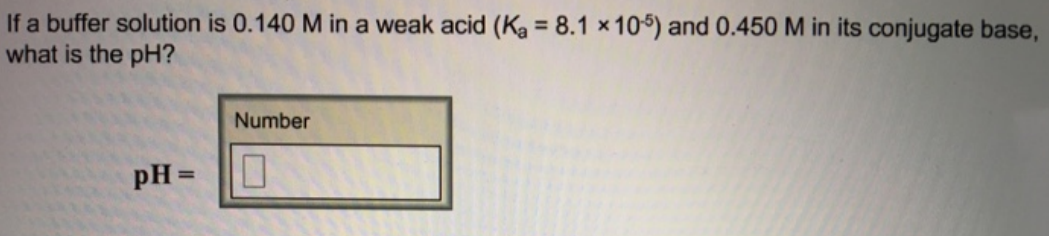# Problem: If a buffer solution is 0.140 M in a weak acid (K_a = 8.1 times 10^-5) and 0.450 M in its conjugate base, what is the pH?

###### FREE Expert Solution
89% (395 ratings)###### Problem Details

If a buffer solution is 0.140 M in a weak acid (K_a = 8.1 times 10^-5) and 0.450 M in its conjugate base, what is the pH?